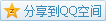# MIT OCW

## 18.02 Multivariable Calculus

### Motion

Recall the cycloid, we use a parametric equation to describe some point $P(x(t),y(t),z(t))$. The vector $\mathbf{r}(t)=\mathbf{OP}=(x(t),y(t),z(t))$ is called position vector, and we can learn about more details when analysing the vector.
Take a cycloid where $t=\theta$ as an example that is $$\mathbf{r}(t)=(t-\sin(t),1-\cos(t)),$$ though it hasn't the third component, but it doesn't lose generality.

#### Velocity

We care about not only the rate at some point but also the direction, in multivariable calculus, a vector can be diffentiated. To get the velocity, we just differentiate the position vector $$\mathbf{v}=\frac{\mathrm{d}\mathbf{r}}{\mathrm{d}t}.$$ In this cycloid case, $$\mathbf{v}=\frac{\mathrm{d}\mathbf{r}}{\mathrm{d}t}=\left(\frac{\mathrm{d}x}{\mathrm{d}t},\frac{\mathrm{d}y}{\mathrm{d}t}\right)=(1-\cos(t),\sin(t)).$$

#### Speed

In some applications, we only care about the rate. The magnitude of velocity becomes speed, in this example that is $$|\mathbf{v}|=\sqrt{(1-\cos(t))^2+\sin^2(t)}=\sqrt{2-2\cos(t)}.$$

### Acceleration

Image you are driving, and you take a tight turn without change of the speed, in the view of single variable calculus, you do not have acceleration. Anyhow, in multivariable calculus, your change of direction is also taken into consideration. Similarly defined like velocity, we have the acceleration $$\mathbf{a}=\frac{\mathrm{d}\mathbf{v}}{\mathrm{d}t}.$$
In this cycloid case, $$\mathbf{a}=\frac{\mathrm{d}\mathbf{v}}{\mathrm{d}t}=(\sin(t),\cos(t)).$$

### Arc Length

If we add velocity continuously, we can get a vector from the start point to the end point. How can we get the arc length during the process? A good idea is to add speed continuously, because speed doesn't have direction. So we have $$\frac{\mathrm{d}s}{\mathrm{d}t}=|\mathbf{v}|.$$
e.g. Length of an arch of cycloid is $\int_0^{2\pi}\sqrt{2-2\cos(t)}\mathrm{d}t$

### Trajectory Unit Tangent Vector

Trajectory unit tangent vector is defined as $\hat{\mathbf{T}}=\frac{\mathbf{v}}{|\mathbf{v}|}$.
And we notice that $$\mathbf{v}=\frac{\mathrm{d}\mathbf{r}}{\mathrm{d}t}=\mathbf{v}=\frac{\mathrm{d}\mathbf{r}}{\mathrm{d}s}\frac{\mathrm{d}s}{\mathrm{d}t},$$ where $\frac{\mathrm{d}s}{\mathrm{d}t}$ is actually $|\mathbf{v}|$, so we have $$\hat{\mathbf{T}}=\frac{\mathrm{d}\mathbf{r}}{\mathrm{d}s}.$$

### Second Law of Kepler

[TO BE CONTINUED]